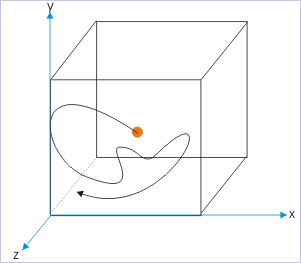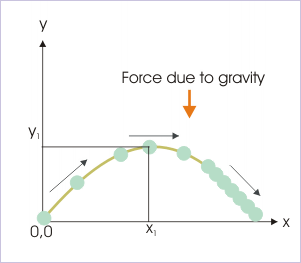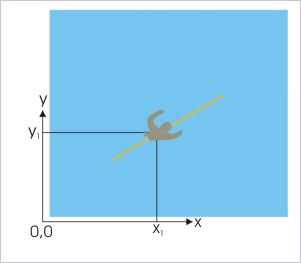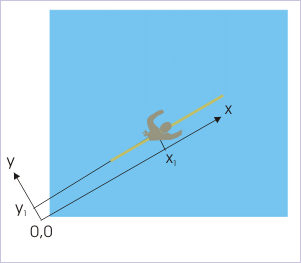# Motion  (Page 4/4)

 Page 4 / 4

Motion in one dimension is rare. This is surprising, because the natural tendency of all bodies is to maintain its motion in both magnitude and direction. This is what Newton’s first law of motion tells us. Logically all bodies should move along a straight line at a constant speed unless it is acted upon by an external force. The fact of life is that objects are subjected to verities of forces during their motion and hence either they deviate from straight line motion or change speed.

Since, real time bodies are mostly non-linear or varying in speed or varying in both speed and direction, we may conclude that bodies are always acted upon by some force. The most common and omnipresent forces in our daily life are the gravitation and friction (electrical) forces. Since force is not the subject of discussion here, we shall skip any further elaboration on the role of force. But, the point is made : bodies generally move in complex manner as they are subjected to different forces.A gas molecule in a container moves randomly under electrostatic interaction with other molecules

Nevertheless, study of motion in one dimension is basic to the understanding of more complex scenarios of motion. The very nature of physical laws relating to motion allows us to study motion by treating motions in different directions separately and then combining the motions in accordance with vector rules to get the overall picture.

A general classification of motion is done in the context of the dimensions of the motion. A motion in space, comprising of three dimensions, is called three dimensional motion. In this case, all three coordinates are changing as the time passes by. While, in two dimensional motion, any two of the three dimensions of the position are changing with time. The parabolic path described by a ball thrown at certain angle to the horizon is an example of the two dimensional motion ( See Figure ). A ball thrown at an angle with horizon is described in terms of two coordinates x and y.A ball thrown at an angle with horizon is described in terms of two coordinates x and y

One dimensional motion, on the other hand, is described using any one of the three coordinates; remaining two coordinates remain constant through out the motion. Generally, we believe that one dimensional motion is equivalent to linear motion. This is not further from truth either. A linear motion in a given frame of reference, however, need not always be one dimensional. Consider the motion of a person swimming along a straight line on a calm water surface. Note here that position of the person at any given instant in the coordinate system is actually given by a pair of coordinate (x,y) values ( See Figure below ).Description of motion requires two coordinate values

There is a caveat though. We can always rotate the pair of axes such that one of it lies parallel to the path of motion as shown in the figure. One of the coordinates, $y{y}_{1}$ is constant through out the motion. Only the x-coordinate is changing and as such motion can be described in terms of x-coordinate alone. It follows then that all linear motion can essentially be treated as one dimensional motion.Choice of appropriate coordinate system renders linear motion as one dimensional motion.

## Kinematics

Kinematics refers to the study of motion of natural bodies. The bodies that we see and deal with in real life are three dimensional objects and essentially not a point object.

A point object would occupy a point (without any dimension) in space. The real bodies, on the other hand, are entities with dimensions, having length, breadth and height. This introduces certain amount of complexity in so far as describing motion. First of all, a real body can not be specified by a single set of coordinates. This is one aspect of the problem. The second equally important aspect is that different parts of the bodies may have path trajectories different to each other.

When a body moves with rotation (rolling while moving), the path trajectories of different parts of the bodies are different; on the other hand, when the body moves without rotation (slipping/ sliding), the path trajectories of the different parts of the bodies are parallel to each other.

In the second case, the motion of all points within the body is equivalent as far as translational motion of the body is concerned and hence, such bodies may be said to move like a point object. It is, therefore, possible to treat the body under consideration to be equivalent to a point so long rotation is not involved.

For this reason, study of kinematics consists of studies of :

1. Translational kinematics
2. Rotational kinematics

A motion can be pure translational or pure rotational or a combination of the two types of motion.

The translational motion allows us to treat a real time body as a point object. Hence, we freely refer to bodies, objects and particles in one and the same sense that all of them are point entities, whose position can be represented by a single set of coordinates. We should keep this in mind while studying translational motion of a body and treating the same as point.

#### Questions & Answers

What are the system of units
Jonah Reply
A stone propelled from a catapult with a speed of 50ms-1 attains a height of 100m. Calculate the time of flight, calculate the angle of projection, calculate the range attained
Samson Reply
58asagravitasnal firce
Amar
water boil at 100 and why
isaac Reply
what is upper limit of speed
Riya Reply
what temperature is 0 k
Riya
0k is the lower limit of the themordynamic scale which is equalt to -273 In celcius scale
Mustapha
How MKS system is the subset of SI system?
Clash Reply
which colour has the shortest wavelength in the white light spectrum
Mustapha Reply
how do we add
Jennifer Reply
if x=a-b, a=5.8cm b=3.22 cm find percentage error in x
Abhyanshu Reply
x=5.8-3.22 x=2.58
sajjad
what is the definition of resolution of forces
Atinuke Reply
what is energy?
James Reply
Ability of doing work is called energy energy neither be create nor destryoed but change in one form to an other form
Abdul
motion
Mustapha
highlights of atomic physics
Benjamin
can anyone tell who founded equations of motion !?
Ztechy Reply
n=a+b/T² find the linear express
Donsmart Reply
أوك
عباس
Quiklyyy
Sultan Reply
Moment of inertia of a bar in terms of perpendicular axis theorem
Sultan Reply
How should i know when to add/subtract the velocities and when to use the Pythagoras theorem?
Yara Reply

### Read also:

#### Get the best Physics for k-12 course in your pocket!

Source:  OpenStax, Physics for k-12. OpenStax CNX. Sep 07, 2009 Download for free at http://cnx.org/content/col10322/1.175
Google Play and the Google Play logo are trademarks of Google Inc.

Notification Switch

Would you like to follow the 'Physics for k-12' conversation and receive update notifications?ByByBy MldelatteByByBy